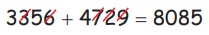Home | | Maths 4th Std | Patterns in numbers: check if it is a multiple of nine

# Patterns in numbers: check if it is a multiple of nine

Cast out nines from a given number to check if it is a multiple of nine.

Patterns in numbers

Cast out nines from a given number to check if it is a multiple of nine.

EXAMPLE

Is 46908 multiple of 9?

46908 = 4 + 6 + 0 + 8

= 18

= 1 + 8

= 9

Let us Know

Any number or combination of digits in that number which add to 9 can be cast out from the given number. Then the sum of remaining digits of the number is divisible by 9 or multiple of 9.

In addition problem, we can check the sum by casting out nines.

EXAMPLE 1

Check the following numbers whether it is a multiple of 9 or not

24689 = 2 + 4 + 6 + 8 = 20 (It's not a multiple of 9.)

9108 = 0 (It's a multiple of 9.)

3165 = 1 + 5 = 6 (It's not a multiple of 9.)

EXAMPLE 2

3356 + 4729 = 80858 + 4 = 21

12 = 21

1 + 2 = 2 + 1

3 = 3

In subtraction problem, we can check the difference by casting out nines method.

(Remember that subtraction is nothing more than addition in reverse).

EXAMPLE

4897 − 2186 = 2711

4897 − 2186 = 2711

19 − 8 = 2

10 − 8 = 2

2 = 2

Let us Know

Think of a two digit number say 52, then subtract the reverse of its digits, 25 from 52.

Difference = 52 − 25 = 27

27 is a multiple of 9.

ActivityTags : Patterns | Term 1 Chapter 3 | 4th Maths , 4th Maths : Term 1 Unit 3 : Patterns
Study Material, Lecturing Notes, Assignment, Reference, Wiki description explanation, brief detail
4th Maths : Term 1 Unit 3 : Patterns : Patterns in numbers: check if it is a multiple of nine | Patterns | Term 1 Chapter 3 | 4th Maths